## Tuesday, March 8, 2011

### Precision Gyro Calibration

Updated version for 2012

Heading accuracy is going to be kind of important and in my data captures thus far, there is a slight discrepancy between the compass and gyro readings.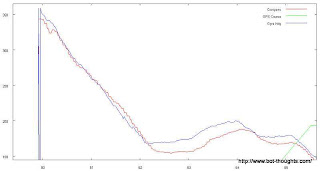Notice the slight discrepancy after long heading changes.
It's possible that the gyro is experiencing some drift when held at a relatively steady rotational rate. It does track the compass heading well in the long run. But I wanted to see if the scale factor was correct or not.

So I decided to make use of my high precision rate gyro calibrator.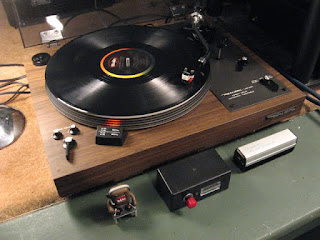High Precision Gyro Rate Calibrator
This is a 1970's Realistic Lab-400 direct drive turntable (it's a surprisingly good-sounding deck, I might add). I would've used my Technics SL-QD33 quartz drive table for greater precision but it's not in such an accessible location.

The Theory

It's really pretty simple. We're talking about a straight line function that, given a voltage, returns a heading rate of change.

Remember y-intercept form?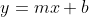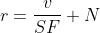Where y is heading rate of change r, x is voltage v, m is actually the inverse of the scale factor SF in degrees/sec, and b is the y-intercept aka the gyro's null value N.

We find the slope (m aka scale factor) by getting two or more x, y points. The turntable can spin at 45 rpm (270°/sec) and 33-1/3 rpm (200°/sec)

Once we know m, we can solve for b. This is all high school algebra stuff. Which is probably why it took me a bit for the memories to come flooding back (hey, it's probably been 25 years! Yes, I am that old).

So let's get our data points.

Data Collection

To get the null value I collected data with the robot perfectly at rest.  To get the 33-1/3 and 45 rpm data, I placed the entire robot, all 5 lbs of it, on a large, empty, inverted can on top of the platter to raise the robot up above where it might rip off my turntable's arm while spinning.

After removing the headshell with my precious cartridge and stylus, I balanced the robot and turned it on to collect data.

But wait. How do I know if the built-in strobe light is precise enough? I don't. And it matters.

I hooked up a high intensity white LED to a breadboard Arduino, and coded up a 50Hz blinker program (tuned with DMM to 3 significant digits).with a 25% duty cycle. The platter is marked for 50Hz and 60Hz.


* ------------
*
* Blinks an LED at 50Hz e.g., for turntable calibration
*/

int ledPin = 8;

void setup()
{
pinMode(ledPin, OUTPUT);      // sets the digital pin as output
}

void loop()
{
digitalWrite(ledPin, HIGH);   // sets the LED on
delayMicroseconds(4960);      // Tests show ~40usec for code exec
digitalWrite(ledPin, LOW);    // sets the LED off
delayMicroseconds(15000);     // total, approx 50Hz
}


Turns out the strobe was off by a percent or two. (Hm, I may have to modify my turntable with a better calibrated strobe...)

I manually spun up the platter and engaged the motor. Since it's a big, powerful motor, it had no problem spinning a 5 lb weight for a few minutes.

Data Analysis

Here are the gyro plots (click to enlarge):

Obviously there's some noise in the signals. I wrote a quick perl script to average the results for the stationary (0°/sec) , 45 rpm (270°/sec) and 33.33 rpm (200°/sec) data captures.

#!/usr/bin/perl

$sum = 0.0;$count = 0;

$GYRO=2; while (<>) { s/[\r\n]+//g; s/^\s+//; @data = split(/\s+/); if ($data[$GYRO] ne 'NaN') {$sum += $data[$GYRO];
$count++; } } print "Sum:$sum  Count $count Avg "; print$sum/$count if ($count != 0);
print "\n";

The output:
• 33.3: Sum: 6743185  Count 2247  Avg 3000.97240765465
• 45: Sum: 10857452  Count 3247  Avg 3343.84108407761
• 0: Sum: 10281588  Count 5072  Avg 2027.12697160883
The ADC used for the gyro is 12 bit and the scale range is 0-5V. To convert the reading to a voltage, multiply by 5.0 ... actually 4.95V according to the DMM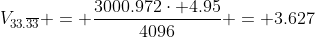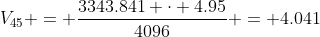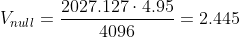Ok! We now have our points, (2.445V, 0°/sec), (4.041V, 270°/sec) and (3.627V, 200°/sec) and we can find the slope aka scale factor.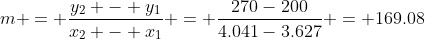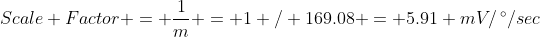Assuming I did all the measurements right, the actual scale factor of the device varies slightly from the 6 mV/°/sec specified in the datasheet. Best not assume...

Double Checking

What if I double check the slope by solving for it using other points? I get 5.886mV (-0.476%) and 5.893 mV/°/sec (-0.353%) versus the 5.91 mV/°/sec.

Not bad. Some of the error may be due to doing null data collection the next day. Perhaps there was some temperature effect.

If the gyro reading had been 2.444V, the scale factors would've been within 0.015% of each other.

Another double check is to solve for the predicted null value from the first two points (45 and 33.3 rpm)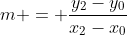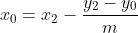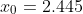The predicted null voltage agrees with the measured voltage to 4 significant digits.

Eliminating Voltage Errors!

EDIT: Coming back to this a few weeks later, and re-reading the datasheet, the null and sensitivity are proportional to voltage.

The datasheet recommends using a ratiometric ADC. Simply, use the same supply for the gyro and the ADC's analog voltage reference. That's just what I did.

Therefore, I don't need to include supply voltage in the calculations above!  Instead of calculating scale factor in terms of volts/°/sec, calculate it in terms of LSBs/°/sec.

That is, find SF in terms of the raw ADC conversion value. Calculate slope from raw ADC values (in bold below).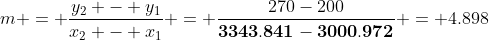Then, in code when it comes time to convert to °/sec, calculate using the bias (null) and SF in raw ADC value.

float gyroRate(unsigned int adc)
{
return ((float) adc - 4027) / 4.898;
}

Other Considerations

Temperature will no doubt affect null and sensitivity somewhat. I'd need to calibrate again for at least two different temperatures to calculate the influence of temperature. I need to see if the additional accuracy is needed by the system or not.

To further reduce error, I could improve the numerical integration approach.

Integrating the Gyro signal more frequently than 20Hz should reduce error accumulation over time.

The integration function can be improved. The simple approach that I'm currently taking is to simply add the fixed gyro voltage to a running sum.  That's a 0th order interpolation function also known as the rectangle (or midpoint) rule.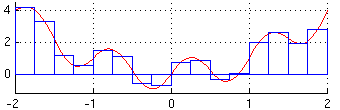Rectangle rule, from Wikipedia
A 1st order interpolation function, the trapezoidal rule, would reduce error and is not computationally intensive.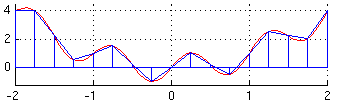Trapezoidal rule, from Wikipedia
There are other interpolation functions. But I can't afford to over-engineer the solution given how much more work I have to do in other areas.

One of my readers, (who has become a trusted adviser!) and blog author of the excellent Guy NXT Door blog, suggests the numerical integration error is negligible compared to other errors. He and I have been working on IMU issues at the same time and comparing notes.

Anyway, no sense in over-engineering with so much else to work on!